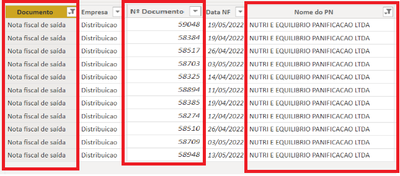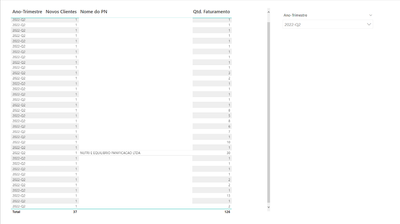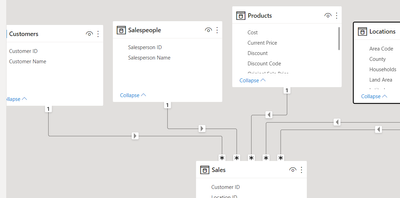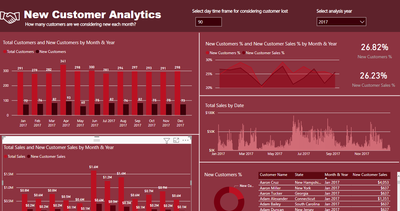cancel
Showing results for
Did you mean:Helper II

## DAX - Sum of the number of notes for each customer

I have a formula that calculates New Customers. Now I need to add the amount of "Outgoing Invoice" for each of them.

``````Novos Clientes =
var vPrimeira_Compra =
CALCULATETABLE(
VALUES(SBOPRODMS[Nome do PN]),
"Data_Primeira_Compra", Calculate(MIN(SBOPRODMS[Data NF]))
),
All(dCalendario),
SBOPRODMS[Documento] = "Nota fiscal de saída"
)
FILTER(
vPrimeira_Compra,
[Data_Primeira_Compra] in VALUES(dCalendario[Date])
)
Return

As an example below I have a customer with 11 invoices.1 ACCEPTED SOLUTIONSuper User

Sorry I thought each row repesents one invoice. Please try

``````Novos Clientes =
VAR vPrimeira_Compra =
CALCULATETABLE (
VALUES ( SBOPRODMS[Nome do PN] ),
"Data_Primeira_Compra", CALCULATE ( MIN ( SBOPRODMS[Data NF] ) )
),
ALL ( dCalendario ),
SBOPRODMS[Documento] = "Nota fiscal de saída"
)
FILTER (
vPrimeira_Compra,
[Data_Primeira_Compra] IN VALUES ( dCalendario[Date] )
)
RETURN
CALCULATE (
DISTINCTCOUNT ( SBOPRODMS[N # Documento] ),
FILTER (
SBOPRODMS,
SBOPRODMS[Nome do PN]
IN SELECTCOLUMNS ( vResultado, "@Nome do PN", [Nome do PN] )
)
)``````
7 REPLIES 7Super User

``````Novos Clientes =
VAR vPrimeira_Compra =
CALCULATETABLE (
VALUES ( SBOPRODMS[Nome do PN] ),
"Data_Primeira_Compra", CALCULATE ( MIN ( SBOPRODMS[Data NF] ) )
),
ALL ( dCalendario ),
SBOPRODMS[Documento] = "Nota fiscal de saída"
)
FILTER (
vPrimeira_Compra,
[Data_Primeira_Compra] IN VALUES ( dCalendario[Date] )
)
RETURN
COUNTROWS (
FILTER (
SBOPRODMS,
SBOPRODMS[Nome do PN]
IN SELECTCOLUMNS ( vResultado, "@Nome do PN", [Nome do PN] )
)
)``````Helper II

The amount of bills is higher, in the example below it would be 11 bills from this customer:Super User

Sorry I thought each row repesents one invoice. Please try

``````Novos Clientes =
VAR vPrimeira_Compra =
CALCULATETABLE (
VALUES ( SBOPRODMS[Nome do PN] ),
"Data_Primeira_Compra", CALCULATE ( MIN ( SBOPRODMS[Data NF] ) )
),
ALL ( dCalendario ),
SBOPRODMS[Documento] = "Nota fiscal de saída"
)
FILTER (
vPrimeira_Compra,
[Data_Primeira_Compra] IN VALUES ( dCalendario[Date] )
)
RETURN
CALCULATE (
DISTINCTCOUNT ( SBOPRODMS[N # Documento] ),
FILTER (
SBOPRODMS,
SBOPRODMS[Nome do PN]
IN SELECTCOLUMNS ( vResultado, "@Nome do PN", [Nome do PN] )
)
)``````Super User

Hi:

The following pattern can work, although it's different in measuring new customers. For instance, you can determine the period of time in which you consider a customer new or lost. In my example below I will use 90 days as this figure. Then you will need just a basic measure that counts total invoices.

Total Invoice = SUM(Table[Invoice No.])

New Customer No. InvoicesSales =
VAR CustomerTM = VALUES( Sales[Customer ID] )
VAR PriorCustomers = CALCULATETABLE( VALUES( Sales[Customer ID] ),
FILTER( ALL( Dates[Date] ),
Dates[Date] > MIN( Dates[Date] ) - 90 &&
Dates[Date] <= MIN( Dates[Date] ) ) )

RETURN
CALCULATE( [Total Invoice],
EXCEPT( CustomerTM, PriorCustomers ) )

To figure new customers count with this method:

New Customers = //calculating which customers within any particular month have purchased but haven't done so for the last x number of days
VAR CustomerTM = VALUES( Sales[Customer ID] )
VAR PriorCustomers = CALCULATETABLE( VALUES( Sales[Customer ID] ),
FILTER( ALL( Dates ),
Dates[Date] > MIN( Dates[Date] ) - 90 &&
Dates[Date] < MIN( Dates[Date] ) ) )

RETURN
COUNTROWS(
EXCEPT( CustomerTM, PriorCustomers ) )

That -90 can be put in a parameter and the user can then select how many days for considering new of lost. That's off topic but available.

I hope this helps!Helper II

Thanks for the answer, but I already tried to implement it with this parameter of 90 days and it doesn't work when using a Date filter.Super User

Hi Diego:

That sounds like your model may need to be star Schema?

The date filters work nicely, so something else might be going on? You can avoid omplicated DAX if you do the data modeling piece. I'll past a somple star schema below and a snipet of a report showing how it all comes together, especially using date filters.Helper II

Can I send the dataset to your email?